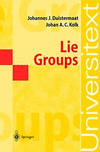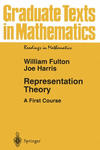MAT 552 Lie groups, Lie algebras and their representations

Place and time MF 09:25 - 10:45, Mon Math 4-130, Fri SBS N-102
Instructor    Sorin Popescu   (office: Math 4-119, tel. 632-8358, e-mail sorin@math.sunysb.edu)

#### Course starts on September 9!

 Course description

This course will cover basic theory of Lie groups and Lie algebras. One of its aims is to provide a brief introduction to modern representation theory by working with the classical examples: the general linear, orthogonal and symplectic Lie groups. The framework will be mainly algebraic, and/or sometimes analytic. We will assume material covered in MAT 530, MAT 531 (Geometry/Topology I-II), MAT 534, MAT 535 (Algebra I-II), as well as certain basic facts from MAT 544 (Analysis). It should however be possible to fill in some of the gaps during the semester.

 Textbook(s)

 The basic textbooks are: Lie Groups, Hans Duistermaat, Johan Kolk, Springer Universitext Representation Theory: A First Course, William Fulton, Joe Harris, Springer, Graduate Texts in Mathematics, Vol. 129 Both books will be on reserve in Math/Phys library. In addition, you may find the following books useful: J. F. Adams, Lectures on Lie Groups - a "classic" J.-P. Serre, Lie algebras and Lie groups - an excellent exposition of the theory of simple Lie algebras Brocker and tom Dieck, Representation of compact Lie groups. A. Knapp, Lie groups beyond an introduction, Birkhauser, 1996 Chevalley, Theory of Lie Groups I.Topics

The following is a tentative list of what we will try to cover in class. Actual material will depend also on the interests of the course participants.

#### Part I. Lie groups

• Basic definitions; examples.
• Linear groups; exponential mapping. Classical groups: SL, SO, Sp, SU.
• Group representations; adjoint representation. Simple and semi-simple representations. Schur lemma
• Compact groups. Haar measure; complete reducibility of representations. Representations of S1.

#### Part II. Lie algebras: basic definitions

• Lie algebra of a Lie group: different definitions.
• Lie theory; exponential mapping, third Lie theorem
• Solvable, simple and semisimple Lie algebras
• Killing form; criterion of semisimplicity. Real forms and complexification.

#### Part III. Structure theory of simple Lie algebras

• Example: sl2 and its representations
• Cartan subalgebra, roots
• Abstract root systems, Cartan matrices, Weyl group. Dynkin diagrams
• Correspondence between root systems and simple Lie algebras

#### Part IV. Representations of simple Lie algebras.

• Representations of sl2, SO(3) and spectrum of the hydrogen atom
• Highest weight representations. Verma modules
• Weyl character formula
• Example: representations of sln

 Homework & Exams

I will assign problems in each lecture, ranging in difficulty from routine to more challenging. There will be also a take-home midterm and a final exam. Course grades will be based on these exams and homework problems (and any other participation).

Sorin Popescu

2002-08-25